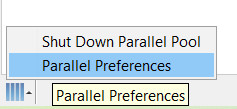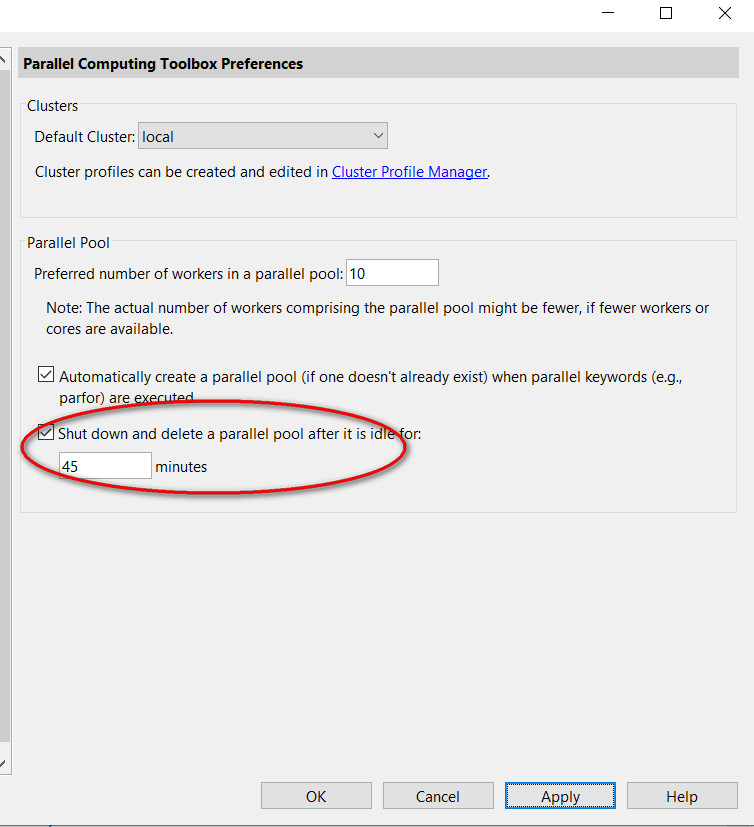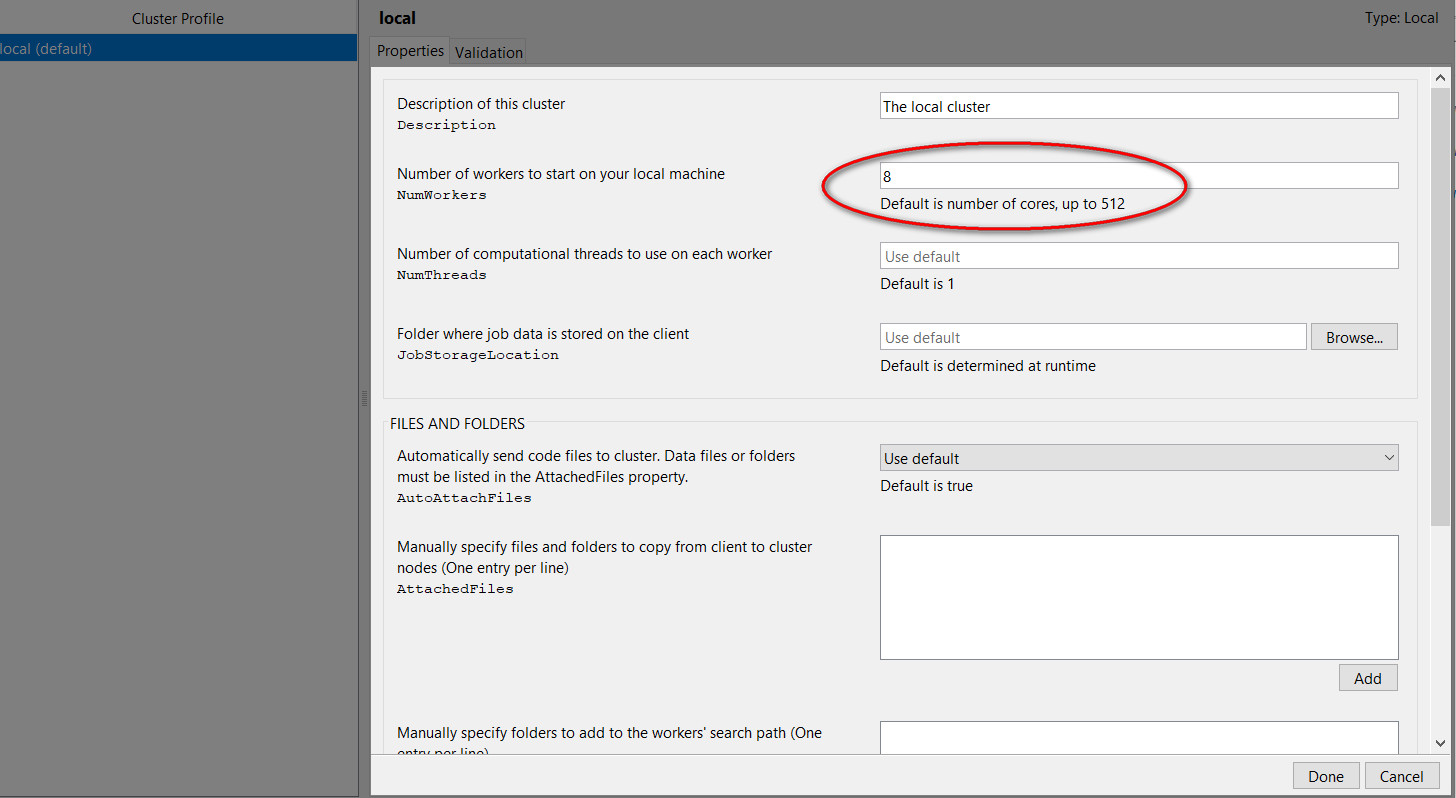# MATLAB循环调用函数使用并行池指南（带非官方实际例子）

Last updated on：3 years ago

# 结果

Got result with index: 1.
Got result with index: 2.
Elapsed time is 131.705283 seconds.
Elapsed time is 143.168543 seconds.

4组输入，也不知道为什么不并行慢了这么多：

Elapsed time is 519.250776 seconds.
Elapsed time is 1362.264277 seconds.

# 并行池的配置# parfeval()函数

F = parfeval(p,fcn,numout,in1,in2,...)
F = parfeval(fcn,numout,in1,in2,...)

p(pool)是并行池得一些其他配置，比如worker数量；fcn是输入的函数名;numout是函数输出参数的数量（可以是0）；in1,in2,in3是输入的参数（有几个输入几个）。

# 并行池的使用

## 官方简单例程

num = 10; % 自己取，测试一下
f(1:num) = parallel.FevalFuture;
for idx = 1:num
f(idx) = parfeval(@magic,1,idx);
end

magicResults = cell(1,num);
for idx = 1:num
[completedIdx,value] = fetchNext(f);
magicResults{completedIdx} = value;
fprintf('Got result with index: %d.\n', completedIdx);
end

%% unparallel
magicResults = cell(1,num);
%% parallel
for idx = 1:num
magicResults{idx} = magic(idx);
end

num = 10;

Elapsed time is 0.264016 seconds.
Elapsed time is 0.000820 seconds.

num = 1000;

Elapsed time is 11.995380 seconds.
Elapsed time is 3.562912 seconds.

num = 1500;

Elapsed time is 28.925654 seconds.
Elapsed time is 12.127613 seconds.

## 工程项目仿真例程

function kuangXiaoHu(FOI,sepparam,geoparam)

geoparam.shape = 2;
FOI = 'ellipsoid'; % field of interest
%% parallel
tic
idex = 0;
f1(1:2) = parallel.FevalFuture;
for theta = [30, 60]
SepParam.theta = theta;
idex = idex + 1;
f1(idex) = parfeval(@kuangXiaoHu,0,FOI,SepParam);
end
%%%%%% 求解
for idx = 1:num
completedIdx = fetchNext(f(idx));
magicResults{completedIdx} = value;
fprintf('Got result with index: %d.\n', completedIdx);
end
toc
%% unparallel
tic
for theta = [30 60]
sepparam.theta = theta;
kuangxiaohu(FOI,SepParam);
end
toc